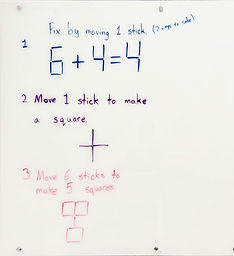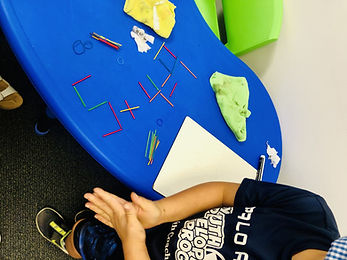## Ms. AJ

### Target 1​

###### Lesson Type:

New

Number Operation

:

Computation

Solve multi-step word problems within 100 (addition, subtraction, multiplication, and division).

###### 1:

Identify the correct arithmetic processes based on the information presented in word problems.

###### 2:

Find the key words in word problems that indicate the correct arithmetic process to use.

###### 3:

Find and use the needed information in a word problem in order to solve.

###### 4:

Write an equation from a word problem with a variable included in the equation. Then solve for the variable.

3rd

###### Vocabulary:

Key Words, Addition, Subtraction, Multiplication, Word Problem

Activities:

Students learned about word problems and how they can be applicable to everyday life.

Students created their own word problems and shared them with the class for other students to first identify key terms and figure out the kind of math they must use, then solve.### Home Exploration

###### Guiding Questions:## Absent Students:

### Target 2

:

###### 1:

Use the given information in a problem to identify the correct answer.

###### 2:

Use the given information to determine which is not the correct answer.

###### 3:

Think outside of the box to solve problems.

###### 4:

Follow given rules to solve problems.

4th

###### Vocabulary:

Out-of-The-Box Thinking

Activities:

Students solved an assortment of "matchstick"/"toothpick" puzzles in which they needed to think of outside of the box ways to solve given problems.### Home Exploration

###### Guiding Questions:### Target 3

:

###### Vocabulary:

Activities:### Home Exploration